International
Tables for
Crystallography
Volume D
Physical properties of crystals
Edited by A. Authier

International Tables for Crystallography (2006). Vol. D, ch. 2.4, pp. 330-331

## Section 2.4.4.2. Scattering cross section

R. Vachera* and E. Courtensa

aLaboratoire des Verres, Université Montpellier 2, Case 069, Place Eugène Bataillon, 34095 Montpellier CEDEX, France
Correspondence e-mail:  rene.vacher@ldv.univ-montp2.fr

#### 2.4.4.2. Scattering cross section

| top | pdf |

The power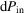, scattered from the illuminated volume V in a solid angle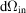, where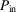and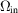are measured inside the sample, is given by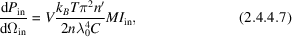where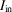is the incident light intensity inside the material,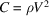is the appropriate elastic constant for the observed phonon, and the factor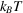results from taking the fluctuation–dissipation theorem in the classical limit for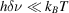(Hayes & Loudon, 1978). The coupling coefficient M is given by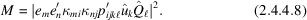In practice, the incident intensity is defined outside the scattering volume,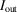, and for normal incidence one can write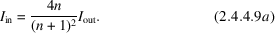Similarly, the scattered power is observed outside as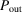, and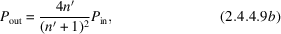again for normal incidence. Finally, the approximative relation between the scattering solid angle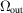, outside the sample, and the solid angle, in the sample, is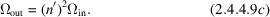Substituting (2.4.4.9a,b,c)in (2.4.4.7), one obtains (Vacher & Boyer, 1972)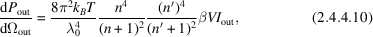where the coupling coefficientis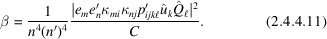In the cases of interest here, the tensoris diagonal,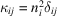without summation on i, and (2.4.4.11)can be written in the simpler form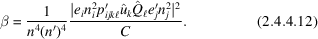### References

Hayes, W. & Loudon, R. (1978). Scattering of light by crystals. New York: Wiley.Google Scholar
Vacher, R. & Boyer, L. (1972). Brillouin scattering: a tool for the measurement of elastic and photoelastic constants. Phys. Rev. B, 6, 639–673.Google Scholar